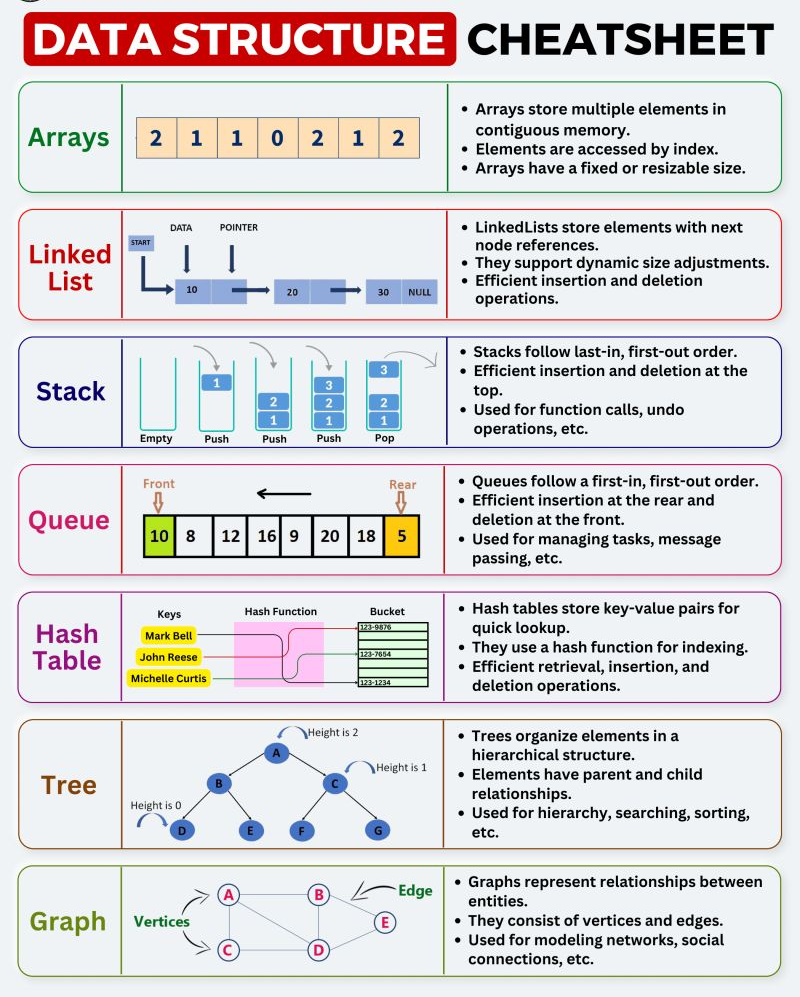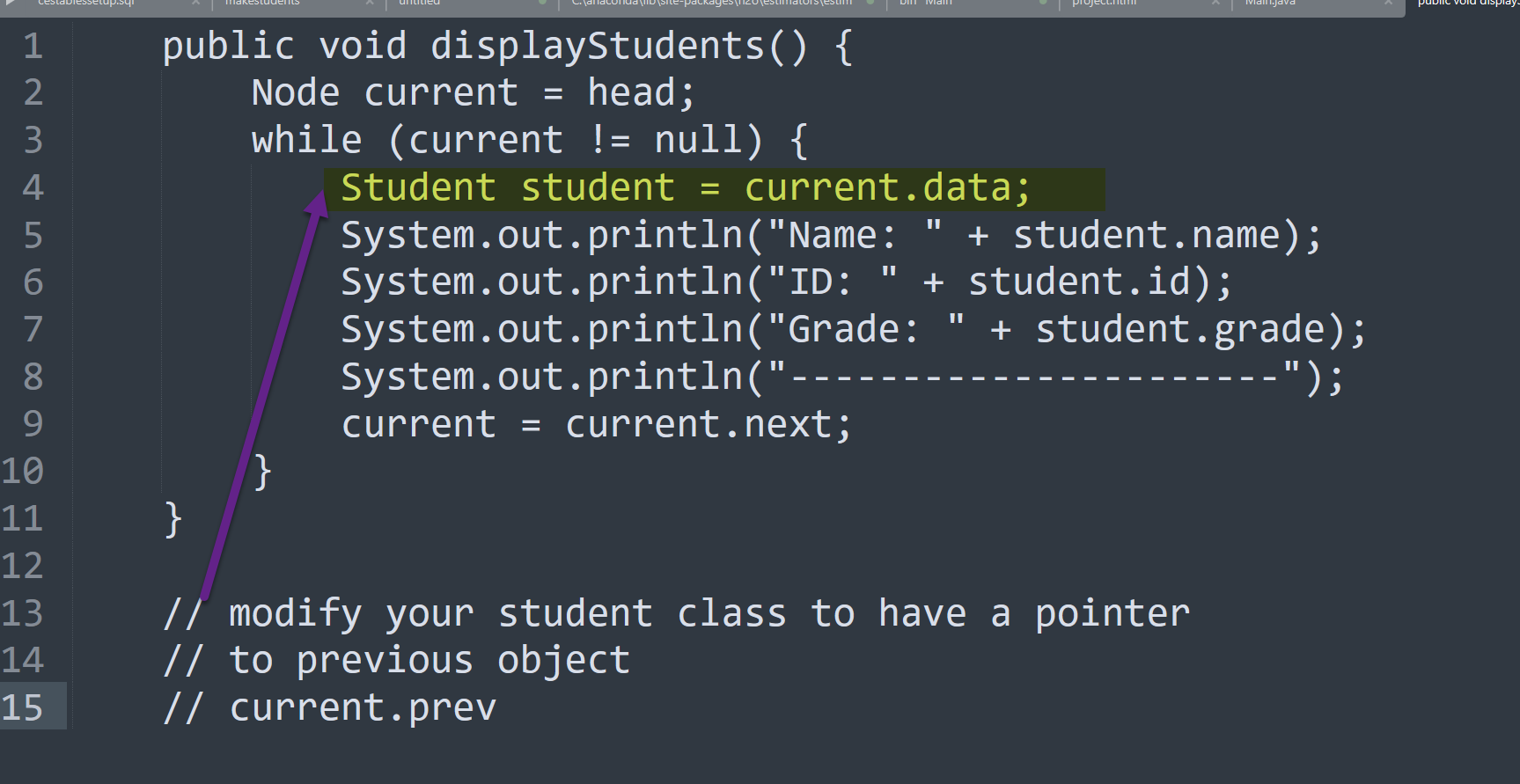Share
Explore

# Assignment 1 Data StructuresAssignment 1 Data Structures

## Data Structures in Java:

In a more advanced Java Programming: you would learn a topic called DSA
Data Structures and Algorithms.
These data structures today are in-memory data structures.
Benefit of in-memory data structures:
→ Our goal as programmers: Think about to push as much of the work as possible to our Data Structures: And let the Data Structures do the processing for us.
Next assigment (2): (Next Week): We will learn how to persist Data into a SQL database.

### How to submit :

Put your code into a GitHub Repository

# Learning Outcome 1:

## Learn how to use GitHUB

First Step in using GitHub.
→ Learn how to set up a GITHUB.com Account.
Use personal email
User NameThese exercises involve storing and retrieving objects from data structures.
The examples are from interesting verticals to make the exercises more engaging.

# Assignment: Understanding Objects in Java through Data Structures

Objective: Explore how Java objects can be stored, manipulated, and retrieved using different types of data structures such as linked lists, stacks, and queues.

## Exercise 1: Implement a Linked List of Student Objects

// Your hand in requirement for exercise 1:
// get the linked list working: with methods to print out the linked list forward and backward
Make a new method: printStudentsInReverse()A linked list is a linear data structure where each element is a separate object. Each element (node) of a list is made up of two items: the data and a reference to the next node.
Task: Create a linked list of Student objects. Each student object should have the following properties: name, id, and grade.
Example:
`public class Student {`` String name;`` int id;`` float grade;``}`
Here's a complete Java code example that demonstrates the creation of a linked list of Student objects with the properties: name, id, and grade. In this example, we'll create a linked list of three students and display their information.

`// Node class represents a single node in the linked list``class Node {`` Student data; // Student object`` Node next; // Reference to the next node`
` public Node(Student student) {`` data = student;`` next = null;`` }``}`
`// Student class represents a student object``class Student {`` String name;`` int id;`` int grade;`
` public Student(String name, int id, int grade) {`` this.name = name;`` this.id = id;`` this.grade = grade;`` }``}`
`// LinkedList class represents the linked list data structure``class LinkedList {`` Node head; // Reference to the first node in the linked list`
` // Method to add a student to the linked list`` public void addStudent(Student student) {`` Node newNode = new Node(student);`` if (head == null) {`` head = newNode;`` } else {`` Node current = head;`` while (current.next != null) {`` current = current.next;`` }`` current.next = newNode;`` }`` }`
` // Method to display the student information in the linked list`` public void displayStudents() {`` Node current = head;`` while (current != null) {`` Student student = current.data;`` System.out.println("Name: " + student.name);`` System.out.println("ID: " + student.id);`` System.out.println("Grade: " + student.grade);`` System.out.println("----------------------");`` current = current.next;`` }`` }``}`

In this example, we create a Student class to represent a student object with name, id, and grade properties. The Node class represents a single node in the linked list, where each node holds a reference to a Student object and the reference to the next node.
The LinkedList class encapsulates the functionality to add students to the linked list and display their information. The addStudent method adds a student by creating a new Node object and appending it to the end of the linked list. The displayStudents method traverses the linked list and prints out the information for each student.
In the main method, we create a LinkedList object, instantiate three Student objects, add them to the linked list using the addStudent method, and finally, display the student information using the displayStudents method.
This example demonstrates the basic functionality of creating a linked list of student objects in Java and displaying their information. You can expand on this code to include additional operations such as searching for a specific student, removing a student from the linked list, etc., based on your requirements.

## Exercise 2: Implement a Stack of Book Objects

A stack is a linear data structure that follows the Last In First Out (LIFO) principle.
Task: Implement a stack of Book objects.
Each book object should have title, author, and isbn properties.
Example:
`public class Book {`` String title;`` String author;`` String isbn;``}`### // Complete Code:

// Here's an example of how you can implement a stack of Book objects
// in Java, along with the data management object that
// provides the push and pop stack handling methods:

import java.util.Stack;

class Book {
String title;
String author;
String isbn;

public Book(String title, String author, String isbn) {
this.title = title;
this.author = author;
this.isbn = isbn;
}
}

class BookStack {
private Stack<Book> stack;

public BookStack() {
stack = new Stack<>();
}

public void push(Book book) {
stack.push(book);
}

public Book pop() {
return stack.pop();
}

public boolean isEmpty() {
return stack.isEmpty();
}